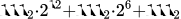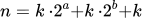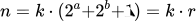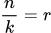# 15 Jul 2015, 15:29

Lately I’ve been solving some problems on CheckiO, a really addictive programming site, and stumbled upon a very interesting golf mission. So, you might ask: “What are golf missions?!”. The idea is to solve the problem using as little code as theoretically possible. Your score is inversely related to code length and transforms a mildly challenging problem into a very addictive one.

One of the most interesting missions is called Ghost Detect. It might not seem special at first, but it gets tricky as solutions get shorter. The mission goal is to write the shortest possible code to recognize if three consecutive signals sent in binary are of the same length. 11000001100011 is a good signal while 111000100011 is a bad one. There is at least one zero between every group of ones. For each character used (less than 200) you earn 1 point. So, how do we do this?

#### 1. Use regex (77 characters, 123 points)

``````import re
recognize=lambda t:len(re.findall(r'^0b(1+)0+\1{1}0+\1{1}\$',bin(t)))
``````

We find and group zeros at the beginning, followed by at least one zero, and then find exactly one occurence of the first group, then some more zeros etc. Not too complicated, but only 123 points. Can we do better?

#### 2. Use the sets, Luke (63 characters, 137 points)

``````import re
recognize=lambda w:len(set(re.findall('1+',bin(w))))<2
``````

Here we combine regex and sets to check how many different groups of repeating ones are present in binary representation.

#### 3. Ditch the regex (57 characters, 143 points)

``````recognize=lambda x:len(set(bin(x)[2:].split('0'))-{''})<2
``````

This is about as far as a “Pythonic” road will take you. Split on zeros, convert to sets, remove empty set to avoid messing with the count and check if less than two.

### Now what?

To further shorten it, we need to try a different approach - binary. In binary, when you multiply by 2, you shift all the bits one position to the left. So, for example, 00010 multiplied by 2 becomes 00100. A valid signal, according to the task, is a number with three groups of ones equally long with zeros inbetween. This actually means it’s made of a number multiplied by a power of two, then the same number was multiplied by some other power of two and added, and then the same number was added again. For example, 111000111000111 is actuallyTherefore, we can write a generalized form for such a number n being equal toThe number should, then, be divisible by both k and r without reminder. First variant that comes to mind is to test if n divided by k equals r. But we have neither k nor r, and the only thing we have to work with is the number given to our function. We have to think of a way to get the number using bit manipulation.

``````n       = 111000111000111
n+1     = 111000111001000
------------------------- XOR
n^n+1   = 000000000001111
n       = 111000111000111
------------------------- AND
n&n^n+1 = 000000000000111
``````

So, now we have k with some bit tricks expressed like n AND n XOR n+1. To find r, we divide n by k and realize it’s basically the original number with sequences of ones reduced to a single 1. We can achieve this too with a little bit manipulation.

``````n       =  111000111000111
n*2     = 1110001110001110
-------------------------- AND
n&n*2   =  110000110000110
n       =  111000111000111
-------------------------- XOR
2*n&n^n =    1000001000001
``````

#### 4. Bit banging:

We are testing for this simple expression.``````recognize=lambda n:n/(n&n^n+1)==2*n&n^n
``````

Down to 39 characters and 161 points. Can we do even better? Suprisingly, yes!

#### 5. Getting rid of k

``````recognize=lambda n:n%(2*n&n^n)==0
``````

If we test divisibility by k, it is easy to construct a number divisible by k which doesn’t satisfy the constraint - e.g. 110011110011. Therefore, we must test divisibility by the other factor, r. Since zeros between the ones are ensured by initial conditions, divisibility by r guarantees the required form.

Our solution is now only 33 characters long (function definition takes 19), and we scored 167 points. There is a trick to get 2 more points which I’m not going to disclose. Can you find it?

#### Can we go above 169?

I don’t think so, but please tell me if you think of a way! :) Hopefully you enjoyed reading about it as much as I’ve enjoyed solving it! If you have another, original solution to this problem feel free to email me and help my curiosity!

Cheers.